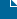## Visualizing My Budget

In Statistical Analysis, Statistics on July 31, 2010 by David Tagged: ,

Given an itemized budget, saved in a spreadsheet as such:You can easily display a picture of how much money you are allocating in each category with a little help from R and ggplot2.The code is such:

library(ggplot2)
#
#Read in Budget File
budget <- read.csv(“budget.csv”, stringsAsFactors = FALSE)
#
#Plot Pie Chart
ggplot(budget, aes(x = factor(1), y = Cost, fill = factor(reorder(Item, Cost*-1)))) +
geom_bar(width = .9, position = “fill”, colour = “black”) +
coord_polar(theta = “y”) +
scale_fill_hue(l=70, c=150)
#
#Plot Stacked Bar Graph
ggplot(budget, aes(x = factor(1), y = Cost, fill = factor(reorder(Item, Cost*-1)))) +
geom_bar(width = .9, position = “fill”, colour = “black”) +
scale_fill_hue(l=70, c=150)